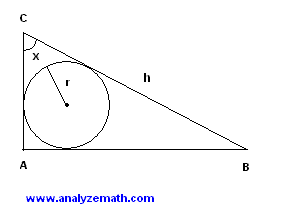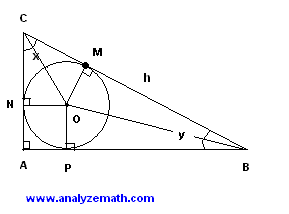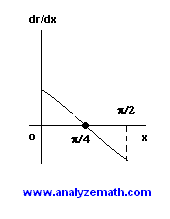# Maximum Radius of Circle Optimization Problem with Solution

Use the derivative to find the size of an angle of a right triangle so that the radius of the circle inscribed is maximum; for a constant hypotenuse.

## Problem

ABC is a right triangle and r is the radius of the inscribed circle.
a) Express r in terms of angle x and the length of the hypotenuse h.
b) Assume that h is constant and x varies; find x for which r is maximum.Solution to Problem:

• a) Let M, N and P be the points of tangency of the circle and the sides of the triangle. OM, ON and OP are perpendicular to CB, CA and AB respectively.• Triangles COM and CON are rigth triangles and have two congruent sides: CO and OM and ON; the two triangles are therefore congruent. We denote the size of angle MCN by x and write
tan(x / 2) = r / CM
• Triangles BOM and BOP are rigth triangles and have two congruent sides: BO and OM and OP; the two triangles are therefore congruent. We denote the size of angle MBP by y and write
tan(y / 2) = r / BM
• Note that
y + x = 90 which gives y / 2 = 45 - x / 2
• Substitute y / 2 by 45 - x / 2 in the equation tan(y / 2) = r / BM to obtain
tan(45 - x / 2) = r / BM
• We now solve the equation tan(x / 2) = r / CM for CM
and solve equation tan(45 - x / 2) = r / BM for BM to obtain

CM = r / tan(x/2) and BM = r / tan(45 - x/2)
• We now use the fact that h = CM + BM to write the equation
h = r / tan(x/2) + r / tan(45 - x/2)
= r [ 1 / tan (x/2) + 1 / tan(45 - x/2) ]
• We now use trigonometric identities to simplify the above equation. The first identity we will use is
tan(45 - x/2) = ( tan(45) - tan(x/2) ) / ( 1 + tan(45)tan(x/2) )
= ( 1 - tan(x/2) ) / ( 1 + tan(x/2) )
• The formula for h is now given by
h = r [ 1 / tan (x/2) + (1 - tan(x/2)) / ( 1 + tan(x/2)) ]
• We now use the identity tan(x/2) = sin(x/2) / cos(x/2) and other identities to rewrite h as follows
h = r [ cos(x/2) / sin(x/2) + (cos(x/2) + sin(x/2)) / (cos(x/2) - sin(x/2)) ]
= r [ 1 / [ (sin(x/2) (cos(x/2) - sin(x/2)) ] ]
• We now solve the above equation for r to obtain
r = h sin(x/2) ( cos(x/2) - sin(x/2) ) = h ( sin(x/2) cos(x/2) - sin 2(x/2))
• We now use the identities sin(x/2)cos(x/2) = (1/2) sin (x) and sin 2(x/2) = 1/2 - (1/2)cos(x) to write r as follows
r = (h / 2) ( sin(x) + cos(x) - 1)
• b) Now that we have calculated r as a function of x, and h assumed constant, let us find the first derivative of r with respect to x
dr / dx = (h / 2) [ cos(x) - sin(x)]
• Let us find a critical points for r by solving the equation dr / dx = 0.
(h / 2) [ cos(x) - sin(x)] = 0
• gives
cos(x) - sin(x) = 0 since h is constant and not equal to 0.
cos(x) = sin(x)
• Square both sides
cos 2(x) = sin 2(x)
cos 2(x) = 1 - cos 2(x)
• Solve for cos x to obtain
cos(x) = + 1/√(2) or - 1/√(2)
• x is a acute angle and therefore the solution to our equation is given by
x = π/4 = 45 degrees.
• The graph of dr/dx is shown below and dr/dx is positive for x < π/4 and negative for x > π/4, therefore r has a maximum at x = π/4 = 45 degrees.• x = 45 degrees is the value of angle ACB for which the radius r is maximum for a given value h of the length of the hypotenuse. Note that in this case triangle ABC is isosceles.

More references on calculus problems